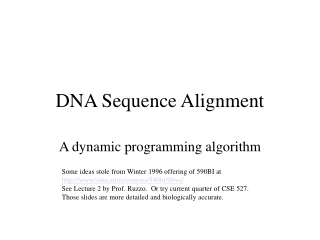DownloadDownload PresentationDNA Sequence Alignment

# DNA Sequence Alignment

Télécharger la présentation## DNA Sequence Alignment

- - - - - - - - - - - - - - - - - - - - - - - - - - - E N D - - - - - - - - - - - - - - - - - - - - - - - - - - -
##### Presentation Transcript

1. DNA Sequence Alignment A dynamic programming algorithm Some ideas stole from Winter 1996 offering of 590BI at http://www/education/courses/590bi/98wi/ See Lecture 2 by Prof. Ruzzo. Or try current quarter of CSE 527. Those slides are more detailed and biologically accurate.

2. DNA Sequence Alignment (aka “Longest Common Subsequence”) • The problem • What is a DNA sequence? • DNA similarity • What is DNA sequence alignment? • Using English words • The Naïve algorithm • The Dynamic Programming algorithm • Idea of Dynamic Programming

3. What is a DNA sequence • DNA: string using letters A,C,G,T • Letter = DNA “base” • e.g. AGATGGGCAAGATA • DNA makes up your “genetic code”

4. DNA similarity • DNA can mutate. • Change a letter • AACCGGTT  ATCCGGTT • Insert a letter • AACCGGTT  ATAACCGGTT • Delete a letter • AACCGGTT  ACCGGTT • A few mutations makes sequences different, but “similar”

5. Why is DNA similarity important • New sequences compared to existing sequences • Similar sequences often have similar function • Most widely used algorithm in computational biology tools • e.g. BLAST at http://www.ncbi.nlm.nih.gov/BLAST/

6. What is DNA sequence alignment? • Match 2 sequences, with underscore ( _ ) wildcards. • Best Alignment  minimum underscores (slight simplification, but okay for 326) • e.g. ACCCGTTT TCCCTTT Best alignment: (3 underscores) A_CCCGTTT _TCCC_TTT

7. Moving to English words zasha ashes zash__a _ashes_

8. Naïve algorithm • Try every way to put in underscores • If it works, and is best so far, record it. • At end, return best solution.

9. Naïve Algorithm – Running Time • Strings size M,N:

10. Dynamic Approach – A table • Table(x,y): best alignment for first x letters of string 1, and first y letters of string 2 • Decide what to do with the end of string, then look up best alignment of remainder in Table.

11. e.g. ‘a’ vs. ‘s’ • “zasha” vs. “ashes”. 2 possibilities for last letters: • (1) match ‘a’ with ‘_’: • best_alignment(“zash”,”ashes”)+1 • (2) match ‘s’ with ‘_’: • best_alignment(“zasha”,”ashe”)+1 •  best_alignment(“zasha”,”ashes”) =min(best_alignment(“zash”,”ashes”)+1, best_alignment(“zasha”,”ashe”)+1)

12. An example

13. Example with solution zasha__ _ash_es

14. Pseudocode (bottom-up) • Given: Strings X,Y , Table[0..x,0..y] • For i=1 to x do • Table[i,0]=i • For j=1 to y do • Table[0,j]=i • i=1, j=1 • While i<=x and j<=y • If X[x]=Y[y] Then • // matches – no underscores • Table[x,y]=Table[x-1,y-1] • Else • Table[x,y]=min(Table[x-1,y],Table[x,y-1])+1 • End If • i=i+1 • If i>x Then • i=1 • j=j+1 • End If

15. Pseudocode (top-down) Given: Strings X,Y , Table[0..x,0..y] BestAlignment (x,y) Compute Table[x-1,y] if necessary Compute Table[x,y-1] if necessary Compute Table[x-1,y-1] if necessary If X[x]=Y[y] Then // matches – no underscores Table[x,y]=Table[x-1,y-1] Else Table[x,y]=min(Table[x-1,y],Table[x,y-1])+1 End If

16. Running time • Every square in table is filled in once • Filling it in is constant time • (n2) squares •  alg is (n2)

17. Albert Q. Dynamic at Whisler mountain Idea of dynamic programming • Re-use expensive computations • Identify critical input to problem (e.g. best alignment of prefixes of strings) • Store results in table, indexed by critical input • Solve cells in table of other cells • Top-down often easier to program Picture from PhotoDisc.com